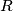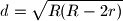IMO Shortlist 1962 problem 6

Kvaliteta:
Avg: 0,0
Težina:
Avg: 0,0
Consider an isosceles triangle. let$R$ be the radius of its circumscribed circle and$r$ be the radius of its inscribed circle. Prove that the distance$d$ between the centers of these two circle isIzvor: Međunarodna matematička olimpijada, shortlist 1962